Question

# How many grams of phosphine (PH3) can form when 34.4 g of phosphorus and 87.7 L...

How many grams of phosphine (PH3) can form when 34.4 g of phosphorus and 87.7 L of hydrogen gas react at STP? P4(s) + H2(g) → PH3(g) (Unbalanced)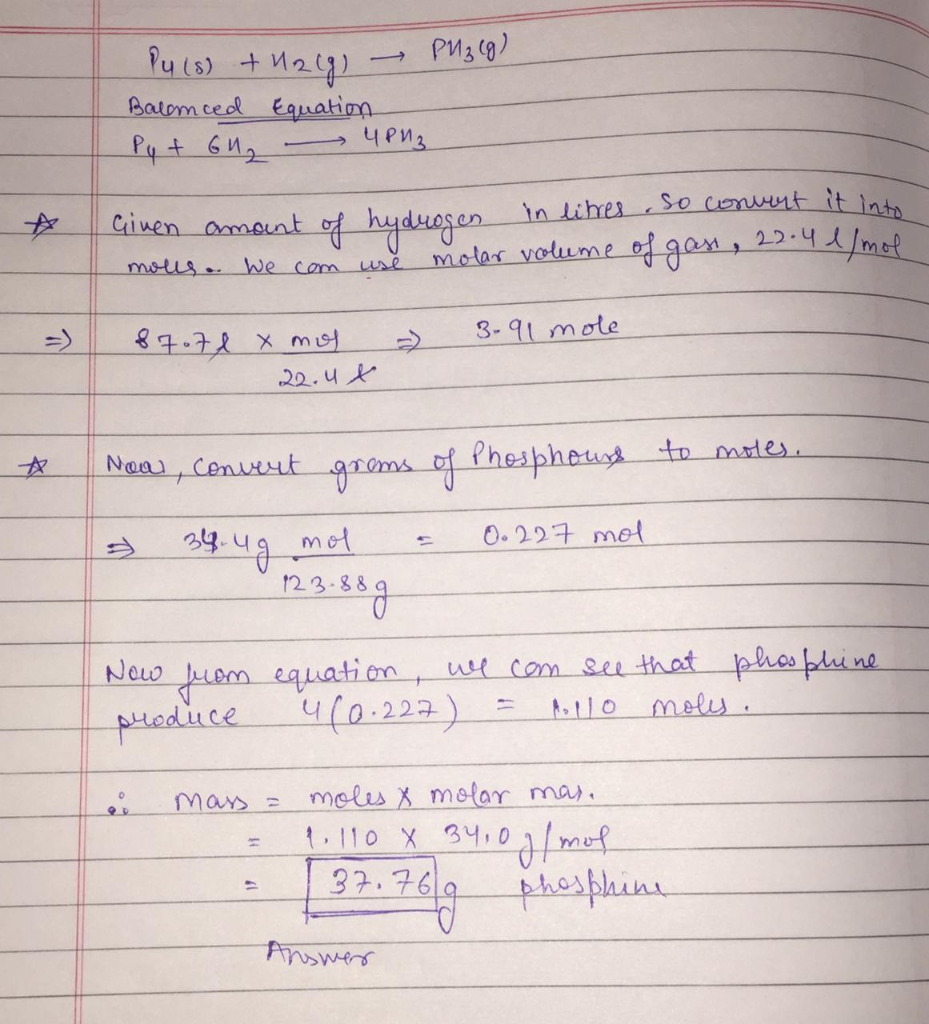#### Earn Coins

Coins can be redeemed for fabulous gifts.

Similar Homework Help Questions
• ### How many grams of phosphine (PH3) can form when 35.0 g of phosphorus and 96.2 L...

How many grams of phosphine (PH3) can form when 35.0 g of phosphorus and 96.2 L of hydrogen gas react at STP? P4(s) + H2(g) → PH3(g) (Unbalanced) ....... g PH3

• ### How many grams of phosphine (PH3) can form when 47.7 g of phosphorus and 95.2 L...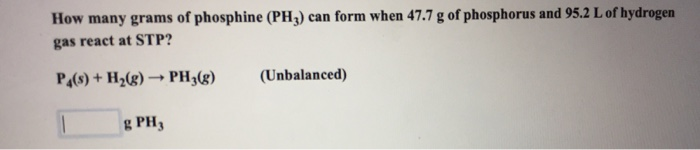How many grams of phosphine (PH3) can form when 47.7 g of phosphorus and 95.2 L of hydrogen gas react at STP? P S)+H2(g)PH,) (Unbalanced) g PH

• ### How many grams of phosphorus react with 37.8 L of O2 at STP to form tetraphosphorus...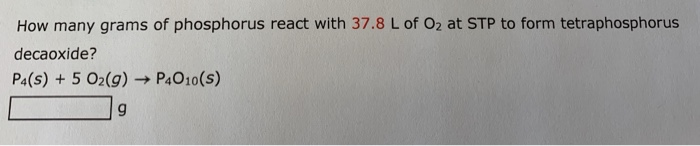How many grams of phosphorus react with 37.8 L of O2 at STP to form tetraphosphorus decaoxide? P4(S) +5 02(g) -P4010(S)

• ### Be sure to answer all parts. The thermal decomposition of phosphine (PH3) into phosphorus and molecular...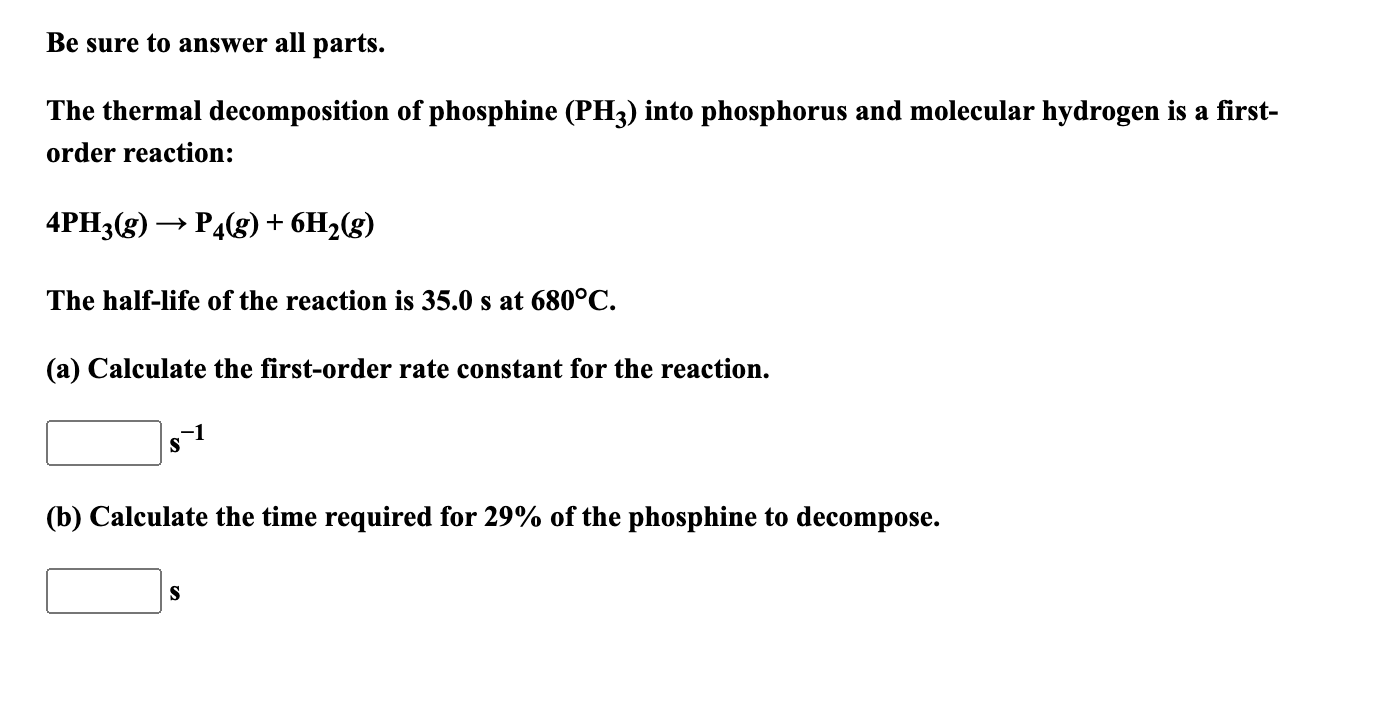Be sure to answer all parts. The thermal decomposition of phosphine (PH3) into phosphorus and molecular hydrogen is a first- order reaction: 4PH3(g) → P4(g) + 6H2(g) The half-life of the reaction is 35.0 s at 680°C. (a) Calculate the first-order rate constant for the reaction. S (b) Calculate the time required for 29% of the phosphine to decompose. S

• ### 10. The thermal decomposition of phosphine (PH3) into phosphorus and molecular hydrogen is a first-order reaction:...

10. The thermal decomposition of phosphine (PH3) into phosphorus and molecular hydrogen is a first-order reaction: 4PH3(g) → P4(g) + 6H2(g) The half-life of the reaction is 35.0 s at 680°C. a) Calculate the first-order rate constant for the reaction: _______ s−1 b) Calculate the time required for 78.0 percent of the phosphine to decompose: ________ s

• ### How many grams of phosphorus (P4) are needed to completely consume 20.3 L of chlorine gas...

How many grams of phosphorus (P4) are needed to completely consume 20.3 L of chlorine gas according to the following reaction at 25 °C and 1 atm? phosphorus (P4) ( s ) + chlorine ( g ) phosphorus trichloride ( l ) _____ grams phosphorus (P4)

• ### the decomposition of phosphine forms phosphorus and hydrogen in the following reaction: 4PH3(g)--> P4(g)+6H2(g) A. express...

the decomposition of phosphine forms phosphorus and hydrogen in the following reaction: 4PH3(g)--> P4(g)+6H2(g) A. express the rate with respect to each of the reactants and oroducts. B. if the instantaneous rate with respect to PH3 is 0.34 M s^-1, what is the instantaneous rate of the reaction? use rate laws not calculus please.

• ### Question 18 (of 18) 5.65 points Ch. Ex. 55- Calculate Mass Formed Using Ideal Gas Law...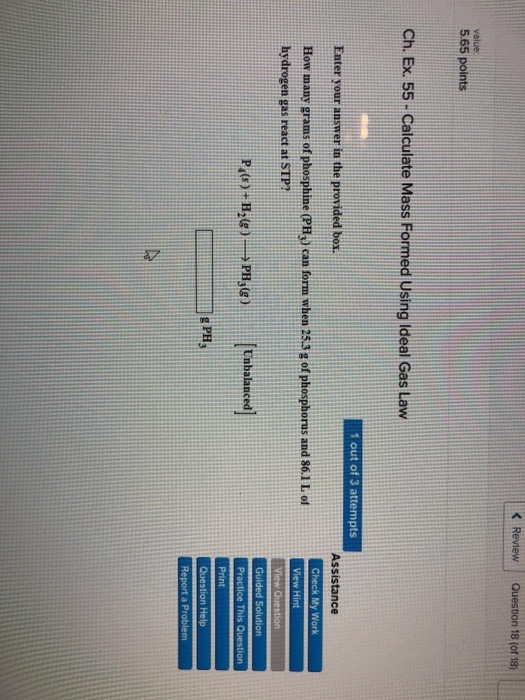Question 18 (of 18) 5.65 points Ch. Ex. 55- Calculate Mass Formed Using Ideal Gas Law 1 out of 3 attempts Enter your answer in the provided box. Assistance How many grams of phosphine (PHy) can form when 253 g of phosphorus and 86.1 L of hydrogen gas react at STP? P-Be PBye) abalanced] g PH

• ### How many moles of phosphorus trichloride may theoretically form when 0.7541 g of phosphorus reacts? (Use...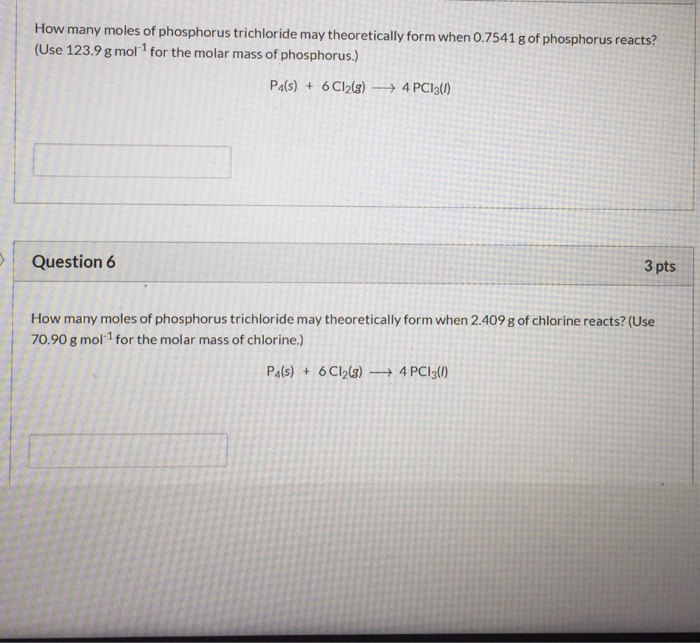How many moles of phosphorus trichloride may theoretically form when 0.7541 g of phosphorus reacts? (Use 123.9 g moll for the molar mass of phosphorus.) P4(s) + 6 C12(g) + 4 PC13(1) Question 6 3 pts How many moles of phosphorus trichloride may theoretically form when 2.409 g of chlorine reacts? (Use 70.90 g mol-1 for the molar mass of chlorine.) P4(s) + 6 C12(8) 4 PC13(1) For the following redox reaction, Fe3O4(s) + H2(g) - 3 Fe(s) + 4H2O(1)...

• ### How many grams of NH3 form when 24.3 L of H2(g) (measured at STP) reacts with...

How many grams of NH3 form when 24.3 L of H2(g) (measured at STP) reacts with N2 to form NH3 according to this reaction? N2(g)+3H2(g)→2NH3(g)